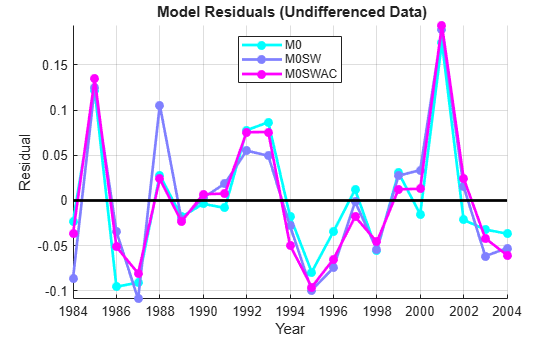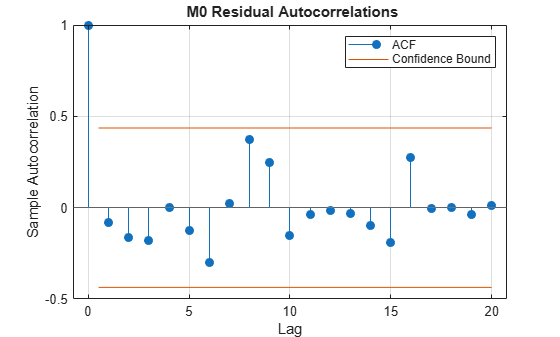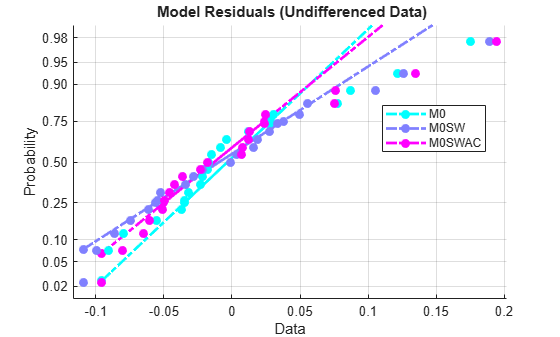## Time Series Regression VI: Residual Diagnostics

This example shows how to evaluate model assumptions and investigate respecification opportunities by examining the series of residuals. It is the sixth in a series of examples on time series regression, following the presentation in previous examples.

### Introduction

The analysis of the credit default data in previous examples in this series has suggested a number of distinct models, using various transformations of the data and various subsets of the predictors. Residual analysis is an essential step for reducing the number of models considered, evaluating options, and suggesting paths back toward respecification. Multiple linear regression (MLR) models with residuals that depart markedly from classical linear model (CLM) assumptions (discussed in the example on "Linear Models") are unlikely to perform well, either in explaining variable relationships or in predicting new responses. Many statistical tests have been developed to assess CLM assumptions about the innovations process, as manifested in the residual series. We examine some of those tests here.

We begin by loading relevant data from the previous example on "Predictor Selection":

load Data_TSReg5

### Residual Plots

The following produces residual plots for each model identified in the previous example, in each of the two model categories (undifferenced and differenced data):

map = cool(3); % Model color map % Undifferenced data: res0 = M0.Residuals.Raw; res0SW = M0SW.Residuals.Raw; res0SWAC = M0SWAC.Residuals.Raw; model0Res = [res0,res0SW,res0SWAC]; figure hold on ax = gca; ax.ColorOrder = map; plot(dates,model0Res,'.-','LineWidth',2,'MarkerSize',20) plot(dates,zeros(size(dates)),'k-','LineWidth',2) hold off legend({'M0', 'M0SW', 'M0SWAC'},'Location','N') xlabel('Year') ylabel('Residual') title('{\bf Model Residuals (Undifferenced Data)}') axis tight grid on% Differenced data: resD1 = MD1.Residuals.Raw; res0SW = MD1SW.Residuals.Raw; res0SWAC = MD1SWA.Residuals.Raw; modelD1Res = NaN(length(dates),3); modelD1Res(2:end,:) = [resD1,res0SW,res0SWAC]; figure hold on ax = gca; ax.ColorOrder = map; plot(dates,modelD1Res,'.-','LineWidth',2,'MarkerSize',20) plot(dates,zeros(size(dates)),'k-','LineWidth',2) hold off legend({'MD1', 'MD1SW', 'MD1SWA'},'Location','N') xlabel('Year') ylabel('Residual') title('{\bf Model Residuals (Differenced Data)}') axis tight grid onFor each model, the residuals scatter around a mean near zero, as they should, with no obvious trends or patterns indicating misspecification. The scale of the residuals is several orders of magnitude less than the scale of the original data (see the example on "Linear Models"), which is a sign that the models have captured a significant portion of the data-generating process (DGP). There appears to be some evidence of autocorrelation in several of the persistently positive or negative departures from the mean, particularly in the undifferenced data. A small amount of heteroscedasticity is also apparent, though it is difficult for a visual assessment to separate this from random variation in such a small sample.

### Autocorrelation

In the presence of autocorrelation, OLS estimates remain unbiased, but they no longer have minimum variance among unbiased estimators. This is a significant problem in small samples, where confidence intervals will be relatively large. Compounding the problem, autocorrelation introduces bias into the standard variance estimates, even asymptotically. Because autocorrelations in economic data are likely to be positive, reflecting similar random factors and omitted variables carried over from one time period to the next, variance estimates tend to be biased downward, toward t-tests with overly optimistic claims of accuracy. The result is that interval estimation and hypothesis testing become unreliable. More conservative significance levels for the t-tests are advised. Robustness of the estimates depends on the extent, or persistence, of the autocorrelations affecting current observations.

The autocorr function, without output arguments, produces an autocorrelogram of the residuals, and gives a quick visual take on the residual autocorrelation structure:

figure autocorr(res0) title('{\bf M0 Residual Autocorrelations}')There is no evidence of autocorrelation outside of the Bartlett two-standard-error bands for white noise, given by the blue lines.

The Durbin-Watson statistic  is the autocorrelation measure most frequently reported in econometric analyses. One reason is that it is easy to compute. For the M0 model:

diffRes0 = diff(res0); SSE0 = res0'*res0; DW0 = (diffRes0'*diffRes0)/SSE0 % Durbin-Watson statistic
DW0 = 2.1474 

Under assumptions of stationary, normally distributed innovations, the statistic is approximately $2\left(1-{\rho }_{1}\right)$, where ${\rho }_{1}$ is the first-order (single lag) autocorrelation estimated by autocorr:

rho0 = autocorr(res0,'NumLags',1); % Sample autocorrelations at lags, 0, 1 DW0Normal = 2*(1-rho0(2))
DW0Normal = 2.1676 

A statistic near 2 provides no evidence of first-order autocorrelation. Appropriate p-values for the statistic are computed by the dwtest method of the LinearModel class:

[pValueDW0,DW0] = dwtest(M0)
pValueDW0 = 0.8943 
DW0 = 2.1474 

The p-value for a null of no first-order autocorrelation is well above the standard 5% critical value.

Econometricians have traditionally relied on a rule of thumb that a Durbin-Watson statistic below about 1.5 is reason to suspect positive first-order autocorrelation. This ad-hoc critical value ignores the dependence on sample size, but it is meant to be a conservative guideline, given the serious consequences of ignoring autocorrelation.

The Durbin-Watson test, though traditionally very popular, has a number of shortcomings. Other than its assumption of stationary, normally distributed innovations, and its ability to detect only first-order autocorrelation, it is very sensitive to other model misspecifications. That is, it is powerful against many alternatives for which the test is not designed. It is also invalid in the presence of lagged response variables (see the example on "Lagged Variables and Estimator Bias").

The Ljung-Box Q-test , implemented by the function lbqtest, tests for "overall" or "portmanteau" lack of autocorrelation. It considers lags up to a specified order L, and so is a natural extension of the first-order Durbin-Watson test. The following tests the M0 residuals for autocorrelation at L = 5, 10, and 15:

[hLBQ0,pValueLBQ0] = lbqtest(res0,'Lags',[5,10,15])
hLBQ0 = 1x3 logical array 0 0 0 
pValueLBQ0 = 1×3 0.8175 0.1814 0.2890 

At the default 5% significance level, the test fails to reject the null of no autocorrelation in each of the extended lag structures. The results are similar for the MD1 model, but much higher p-values indicate even less evidence for rejecting the null:

[hLBQD1,pValueLBQD1] = lbqtest(resD1,'Lags',[5,10,15])
hLBQD1 = 1x3 logical array 0 0 0 
pValueLBQD1 = 1×3 0.9349 0.7287 0.9466 

The Q-test also has its shortcomings. If L is too small, the test will not detect higher-order autocorrelations. If it is too large, the test will lose power, since a significant correlation at any lag may be washed out by insignificant correlations at other lags. Also, the test is powerful against serial dependencies other than autocorrelation.

Another shortcoming of the Q-test is that the default chi-square distributions used by the test are asymptotic, and can produce unreliable results in small samples. For ARMA(p, q) models, for which the test was developed, more accurate distributions are obtained if the number of degrees of freedom is reduced by the number of estimated coefficients, p + q. This limits the test, however, to values of L greater than p + q, since the degrees of freedom must be positive. Similar adjustments can be made for general regression models, but lbqtest does not do so by default.

Another test for "overall" lack of autocorrelation is a runs test, implemented by the function runstest, which determines if the signs of the residuals deviate systematically from zero. The test looks for long runs of either the same sign (positive autocorrelation) or alternating signs (negative autocorrelation):

[hRT0,pValueRT0] = runstest(res0)
hRT0 = 0 
pValueRT0 = 0.2878 

The test fails to reject the null of randomness in the residuals of the M0 model.

Autocorrelated residuals may be a sign of a significant specification error, in which omitted, autocorrelated variables have become implicit components of the innovations process. Absent any theoretical suggestions of what those variables might be, the typical remedy is to include lagged values of the response variable among the predictors, at lags up to the order of autocorrelation. Introducing this kind of dynamic dependence into the model, however, is a significant departure from the static MLR specification. Dynamic models present a new set of considerations relative to the CLM assumptions, and are considered in the example on "Lagged Variables and Estimator Bias."

### Heteroscedasticity

Heteroscedasticity occurs when the variance of the predictors and the innovations process produce, in aggregate, a conditional variance in the response. The phenomenon is commonly associated with cross-sectional data, where systematic variations in measurement error can occur across observations. In time series data, heteroscedasticity is more often the result of interactions between model predictors and omitted variables, and so is another sign of a fundamental misspecification. OLS estimates in the presence of heteroscedasticity exhibit virtually identical problems to those associated with autocorrelation; they are unbiased, but no longer have minimum variance among unbiased estimators, and standard formulas for estimator variance become biased. However, Monte Carlo studies suggest that the effects on interval estimation are usually quite minor . Unless heteroscedasticity is pronounced, distortion of the standard errors is small, and significance tests are largely unaffected. With most economic data, the effects of heteroscedasticity will be minor compared to the effects of autocorrelation.

Engle's ARCH test , implemented by the archtest function, is an example of a test used to identify residual heteroscedasticity. It assesses the null hypothesis that a series of residuals ${r}_{t}$ exhibits no conditional heteroscedasticity (ARCH effects), against the alternative that an ARCH(L) model

${r}_{t}^{2}={a}_{0}+{a}_{1}{r}_{t-1}^{2}+...+{a}_{L}{r}_{t-L}^{2}+{\zeta }_{t},$

describes the series with at least one nonzero ${a}_{k}$ for $k=0,...,L$. Here ${\zeta }_{t}$ is an independent innovations process. The residuals in an ARCH process are dependent, but not correlated, so the test is for heteroscedasticity without autocorrelation.

Applying the test to the M0 residual series with lags L = 5, 10, and 15 gives:

[hARCH0,pARCH0] = archtest(res0,'Lags',[5,10,15])
hARCH0 = 1x3 logical array 0 0 0 
pARCH0 = 1×3 0.4200 0.3575 0.9797 

The test finds no evidence of heteroscedasticity in the residuals. For the MD1 model the evidence is even weaker:

[hARCHD1,pARCHD1] = archtest(resD1,'Lags',[5,10,15])
hARCHD1 = 1x3 logical array 0 0 0 
pARCHD1 = 1×3 0.5535 0.4405 0.9921 

### Distribution

The assumption that the innovations process is normally distributed is not required by the Gauss-Markov theorem, but it is necessary for confidence intervals to be constructed using standard techniques, and for t and F tests to provide accurate assessments of predictor significance. The assumption is especially important in small samples, where the Central Limit theorem cannot be relied upon to provide approximately normal distributions of estimates, independent of the distribution of innovations.

The usual justification for the normality assumption is that innovations are the sum of inherent stochasticity plus all of the variables omitted from the regression. The Central Limit theorem says that this sum will approach normality as the number of omitted variables increases. However, this conclusion depends on the omitted variables being independent of each other, and this is often unjustified in practice. Thus, for small samples, regardless of the results on autocorrelation and heteroscedasticity, checking the normality assumption is an important component of accurate specification.

A normal probability plot of the residual series gives a quick assessment:

figure hNPlot0 = normplot(model0Res); legend({'M0', 'M0SW', 'M0SWAC'},'Location','Best') title('{\bf Model Residuals (Undifferenced Data)}') set(hNPlot0,'Marker','.') set(hNPlot0([1 4 7]),'Color',map(1,:)) set(hNPlot0([2 5 8]),'Color',map(2,:)) set(hNPlot0([3 6 9]),'Color',map(3,:)) set(hNPlot0,'LineWidth',2) set(hNPlot0,'MarkerSize',20)figure hNPlotD1 = normplot(modelD1Res); legend({'MD1', 'MD1SW', 'MD1SWA'},'Location','Best') title('{\bf Model Residuals (Differenced Data)}') set(hNPlotD1,'Marker','.') set(hNPlotD1([1 4 7]),'Color',map(1,:)) set(hNPlotD1([2 5 8]),'Color',map(2,:)) set(hNPlotD1([3 6 9]),'Color',map(3,:)) set(hNPlotD1,'LineWidth',2) set(hNPlotD1,'MarkerSize',20)The plots show empirical probability versus residual value. Solid lines connect the 25th and 75th percentiles in the data, then are extended by dashed lines. The vertical scale is nonlinear, with the distance between tick marks equal to the distance between normal quantiles. If the data fall near the line, the normality assumption is reasonable. Here, we see an apparent departure from normality for data with large residuals (again, especially in the undifferenced data), indicating that the distributions may be skewed. Clearly, removal of the most influential observations, considered in the example on "Influential Observations," would improve the normality of the residuals.

It is a good idea to confirm any visual analysis with an appropriate test. There are many statistical tests for distributional assumptions, but the Lilliefors test, implemented by the lillietest function, is a normality test designed specifically for small samples:

[hNorm0,pNorm0] = lillietest(res0)
hNorm0 = 1 
pNorm0 = 0.0484 

At the default 5% significance level, the test rejects normality in the M0 series, but just barely. The test finds no reason to reject normality in the MD1 data:

s = warning('off','stats:lillietest:OutOfRangePHigh'); % Turn off small statistic warning [hNormD1,pNormD1] = lillietest(resD1)
hNormD1 = 0 
pNormD1 = 0.5000 
warning(s) % Restore warning state

The statistic is at the edge of the table of critical values tabulated by lillietest, and the largest p-value is reported.

A common remedy for nonnormality is to apply a Box-Cox transformation to the response variable . Unlike log and power transformations of predictors, which are primarily used to produce linearity and facilitate trend removal, Box-Cox transformations are designed to produce normality in the residuals. They often have the beneficial side effect of regularizing the residual variance.

Collectively, Box-Cox transformations form a parameterized family with log and standardized power transformations as special cases. The transformation with parameter $\lambda$ replaces the response variable ${y}_{t}$ with the variable:

${{y}_{t}}^{\left(\lambda \right)}=\frac{{{y}_{t}}^{\lambda }-1}{\lambda }$

for $\lambda \ne 0$. For $\lambda =0$, the transformation is given by its limiting value, log(${y}_{t}$).

The boxcox function in Financial Toolbox finds the parameter ${\lambda }_{0}$ that maximizes the normal loglikelihood of the residuals. To apply the function to the IGD data in y0, it is necessary to perturb the zero default rates to make them positive:

alpha = 0.01; y0(y0 == 0) = alpha; % y0BC = boxcox(y0); % In Financial Toolbox y0BC = [-3.5159 -1.6942 -3.5159 -3.5159 -1.7306 -1.7565 -1.4580 -3.5159 -3.5159 -2.4760 -2.5537 -3.5159 -2.1858 -1.7071 -1.7277 -1.5625 -1.4405 -0.7422 -2.0047 -3.5159 -2.8346];

The transformation is sensitive to the value of alpha, which adds a level complication to the analysis. A Lilliefors test, however, confirms that the transform has the desired effect:

M0BC = fitlm(X0,y0BC); res0BC = M0BC.Residuals.Raw; [hNorm0BC,pNorm0BC] = lillietest(res0BC)
hNorm0BC = 0 
pNorm0BC = 0.4523 
warning(s) % Restore warning state

Since the evidence for nonnormality in the original residual series is slight, we do not pursue a fine-tuning of the Box-Cox transformation.

### Summary

The fundamental purpose of residual analysis is to check CLM assumptions and look for evidence of model misspecification. Patterns in the residuals suggest opportunities for respecification to obtain a model with more accurate OLS coefficient estimates, enhanced explanatory power, and better forecast performance.

Different models can exhibit similar residual characteristics. If so, alternative models may need to be retained and further assessed at the forecasting stage. From a forecasting perspective, if a model has successfully represented all of the systematic information in the data, then the residuals should be white noise. That is, if the innovations are white noise, and the model mimics the DGP, then the one-step-ahead forecast errors should be white noise. Model residuals are in-sample measures of these out-of-sample forecast errors. Forecast performance is discussed in the example on "Forecasting."

The problems of OLS estimation associated with non-white innovations, coupled with the limited options for respecifying many economic models, has led to the consideration of more robust heteroscedasticity and autocorrelation consistent (HAC) estimators of variance, such as the Hansen-White and Newey-West estimators, which eliminate asymptotic (though not small-sample) bias. Revised estimation techniques, such as generalized least squares (GLS), have also been developed for estimating coefficients in these cases. GLS is designed to give lower weight to influential observations with large residuals. The GLS estimator is BLUE (see the example on "Linear Models"), and equivalent to the maximum likelihood estimator (MLE) when the innovations are normal. These techniques are considered in the example on "Generalized Least Squares & HAC Estimators."

### References

 Bohrnstedt, G. W., and T. M. Carter. "Robustness in Regression Analysis." In Sociological Methodology, H. L. Costner, editor, pp. 118-146. San Francisco: Jossey-Bass, 1971.

 Box, G. E. P., and D. R. Cox. "An Analysis of Transformations". Journal of the Royal Statistical Society. Series B, Vol. 26, 1964, pp. 211-252.

 Durbin, J. and G.S. Watson. "Testing for Serial Correlation in Least Squares Regression." Biometrika. Vol. 37, 1950, pp. 409-428.

 Engle, R. F. "Autoregressive Conditional Heteroscedasticity with Estimates of the Variance of United Kingdom Inflation." Econometrica. Vol. 50, 1982, pp. 987-1007.

 Ljung, G. M. and G. E. P. Box. "On a Measure of Lack of Fit in Time Series Models." Biometrika. Vol. 65, 1950, pp. 297-303.